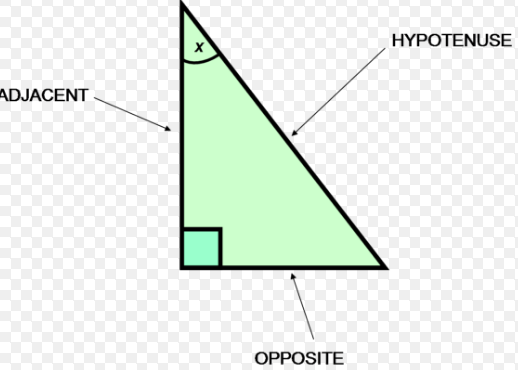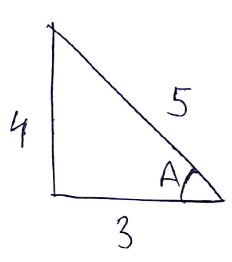# If an angle A of a ΔABC satisfies  , then the roots of the quadratic equation,  are : Option 1) secA, cotA Option 2) sinA, secA Option 3) secA, tanA Option 4) tanA, cosA

A Anuj Pandey
H Himanshu

AS we have learned

Trigonometric Ratios of Functions -

- whereinon solving

so

Option 1)

secA, cotA

This is incorrect

Option 2)

sinA, secA

This is incorrect

Option 3)

secA, tanA

This is correct

Option 4)

tanA, cosA

This is incorrect

Exams
Articles
Questions